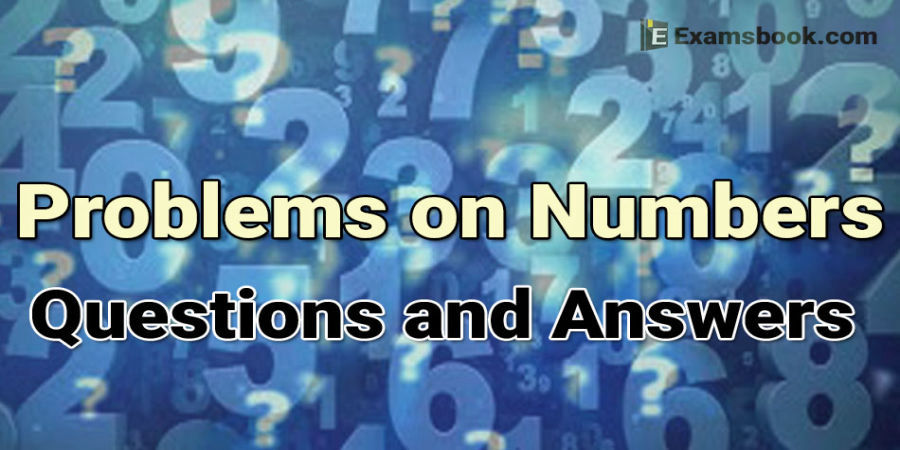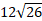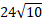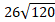• Save

# Problem on number aptitude question and answers

4 months ago 2.9K ViewsProblem on number questions have been asked in many competitive exams and there are strong chances to ask number questions again in SSC, Bank and other competitive exams. Students should prepare for exams by practicing problem on number questions and answers.

Problem on number questions are important and 1-2 questions asked in exams every year, try to solve Problem on number aptitude questions and answers for better results in upcoming competitive exams.

Problems are part of our life but every problem has a solution. So, here you can learn the numbers problems with solutions by one click for better the preparation of your competitive exams as well as Number Series Problems with Solutions.

## Important Problem on number questions and Answers: Aptitude Questions

1. One-fifth of a number is equal toof another number. If 35 is added to the first number, it becomes four times of the second number. The second number is

(A) 30

(B) 40

(C) 50

(D) 70

Ans .   B

2. If the sum of two number is 33 and their difference is 15, the smaller number is:

(A) 9

(B) 13

(C) 16

(D) 18

Ans .   A

3. The product of two number is 192 and the sum of these two number is 28. What is the smaller of these two number?

(A) 12

(B) 15

(C) 18

(D) 20

Ans .  A

4. Two numbers differ by 5. If their product is 336, then the sum of the two number is:

(A) 23

(B) 29

(C) 37

(D) 52

Ans .   C

5. They product of two number is 9375 and the quotient, when the larger one is divided by the smaller, is 15. The sum of the number is:

(A) 385

(B) 395

(C) 400

(D) 425

Ans .   C

6. The sum of three consecutive number is 87. The greatest among these three number is:

(A) 27

(B) 29

(C) 28

(D) 30

Ans .   D

7. The sum of four consecutive even integers is 1284. The greatest of them is :

(A) 318

(B) 320

(C) 324

(D) 328

Ans .   C

8. The product of three consecutive even numbers when divided by 8 is 720. The product of their square roots is :

(A)(B)(C)(D) None of these

Ans .   B

Ask me in the comment section, If you face any problem while solving number questions. Visit next page for more Problem on number questions and answers.

## Important Problem on number questions and Answers: Aptitude Questions

9. What is the sum of two consecutive even number, the difference of whose squares is 84?

(A) 43

(B) 38

(C) 42

(D) 46

Ans .   C

10. There are two number such that the sum of twice the first and thrice the second is 39, while the sum of thrice the first and twice the second is 36. The larger of the two is:

(A) 7

(B) 8

(C) 9

(D) 10

Ans .   C

11. In a two digits number, if it is known that is its units digit exceeds its ten’s digit by 2 and that the product of the given number and the sum of its digits is equal to 144, then the number is:

(A) 24

(B) 27

(C) 42

(D) 44

Ans .   A

12. The sum of the digits of a two-digit number is 9 less than the number. Which of the following digits is at unit’s place of the number?

(A) 1

(B) 2

(C) 3

(D) 4

Ans .   D

13. The difference between a two-digit number and the number obtained by interchanging the two digits is 63. Which is the smaller of the two number?

(A) 29

(B) 31

(C) 70

(D) Cannot be determined

Ans .   D

14. The sum of two number is 25 and their difference is 13. Find their product.

(A) 105

(B) 114

(C) 316

(D) 225

Ans .   B

15. The sum of two number is 40 and their difference is 4. The ratio of the number is:

(A) 11: 9

(B) 13: 11

(C) 21: 19

(D) 22: 7

Ans .   A

16. The difference between two integers is 5. Their product is 500. Find the numbers.

(A) 16,20

(B) 20, 25

(C) 30, 25

(D) 21,26

Ans .   B

Ask me in the comment section, If you face any problem while solving number questions. Visit next page for more Problem on number questions and answers.

## Important Problem on number questions and Answers: Aptitude Questions

17. Two different natural number are such that their product is less than their sum. One of the number must be:

(A) 1

(B) 2

(C) 3

(D) 4

Ans .   A

18. The difference between two number is 1365. When the larger number is divided by the smaller one, the quotient is 6 and the remainder is 15. The smaller number is :

(A) 250

(B) 270

(C) 290

(D) 300

Ans .   B

19. Three times the first of three consecutive odd integers are 3 more than twice the third. The third integer is

(A) 11

(B) 9

(C) 13

(D) 15

Ans .   D

20. The sum of three consecutive odd number is 20 more than the first of these numbers. What is the middle number?

(A) 8

(B) 9

(C) 11

(D) 13

Ans .   B

21. The sum of three consecutive multiples of 3 is 72. What is the largest number?

(A) 22

(B) 26

(C) 27

(D) 32

Ans .   C

22. The sum of the squares of three consecutive natural number is 2030. What is the middle number?

(A) 24

(B) 26

(C) 29

(D) 30

Ans .   B

23. In a two-digit number, the digit in the unit’s place is four times the digit in ten’s place and sum of the digits is equal to 10. What is the number

(A) 15

(B) 43

(C) 53

(D) 82

(E) None of these

Ans .   E

24. A number consist of two digits. If the digits interchange places and the new number is added to the original number, then the resulting number will be divisible by:

(A) 6

(B) 5

(C) 8

(D) 11

Ans .   D

Ask me in the comment section, If you face any problem while solving number questions. Visit next page for more Problem on number questions and answers.

## Important Problem on number questions and Answers: Aptitude Questions

25. The difference between a two-digit number and the number obtained by interchanging the position of its digits is 36. What is the difference between the two digits of that number?

(A) 5

(B) 4

(C) 9

(D) None of these

Ans .   B

26. The sum of the digits of a two-digit number isof the difference between the number and the number obtained by interchanging the position of the digits. What is definitely the difference between the digits of that number?

(A) 6

(B) 8

(C) 11

(E) None of these

Ans .   D

Ask me in the comment section, If you face any problem while solving number questions. Visit next page for more Problem on number questions and answers.